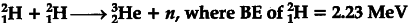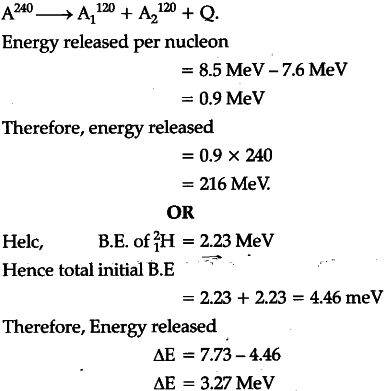# A nucleus with mass numbers

A nucleus with mass number A = 240 and BE/A = 7.6 MeV breaks into two fragments each of A = 120 with BE/A = 8.5 MeV. Calculate the released energy.
OR
Calculate the energy in fusion reaction:and of
_{ 2 }^{ 3 }{ He=7.73\quad Mev. }What is BE/A plz explain if possible thank you